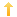## PowerBuilder – Setting column value without looping through all rows.

Posted on Thursday, March 3rd, 2011 at 8:00 pm in

A question came up on the TekTips website about setting a column in all the rows in a datawindow to the same value without looping through. In this case the writer was loading data from over one hundred Excel files and wanted to track the filename of where the data came from. At first I thought you could simply put an expression to modify the data in a column but you cannot. Then I thought you could set up a computed column and modify the expression but you cannot do a rowscopy from a computed column. Then I came up with the following.

Since the data is being imported via the importfile method, I needed to set the initial value of a column to the filename within the ‘working’ or ‘temp’ datawindow which the data is initially loaded into. After defining the external datawindow with the number of data columns equal to my import files, I added a row to the column definitions in the table section. This object I called “d_excelloadtemp”.

` column=(type=char(255) updatewhereclause=yes name=file_name dbname="file_name" )`

In my case this was the fifth line in that section. This is important since when defining the column for the modify, you need to give it an ID value which is the same as the order in which it appears in the table section, in this case 5.

The permanent datawindow object has all five columns defined with the ‘file_name’ column being the same as the added one in the ‘temp’ datawindow object. In fact, I pulled its visual definition syntax, which is used in the ‘create column’ modify statement, from the source of this datawindow (called “d_excelload”).

The code is in the clicked event of the load button.

```integer li_rc
long ll_rc
string ls_filename, ls_msg, ls_modify
// first file to process
ls_filename = 'c:\temp\inventoryA.csv'

// the modify is taken from the datawindow object source.  Remember to put a tilde in front
// of any double quotes.

ls_msg = dw_2.modify(ls_modify)

// Below is where we load two files.  If you plan on processing many, put this in a loop
// set the initial value of the new column
ls_modify = "file_name.initial='" + ls_filename + "'"
ls_msg = dw_2.modify(ls_modify)
// import rows
ll_rc = dw_2.importfile(ls_filename)
// copy the data to the permanent datawindow
li_rc = dw_2.rowscopy( 1, 9999, Primary!, dw_1, 9999, Primary!)

dw_2.reset()
// next file
ls_filename = 'c:\temp\inventoryB.csv'
// modify the value of the dynamic column
ls_modify = "file_name.initial='" + ls_filename + "'"
ls_msg = dw_2.modify(ls_modify)

ll_rc = dw_2.importfile(ls_filename)
// copy again to the permanent datawindow
li_rc = dw_2.rowscopy( 1, 9999, Primary!, dw_1, 9999, Primary!)```

Export files can be found excellload. This is in PB11.5.1

You might also be interested in
Tags: